# Learn Functions in Python

0
3340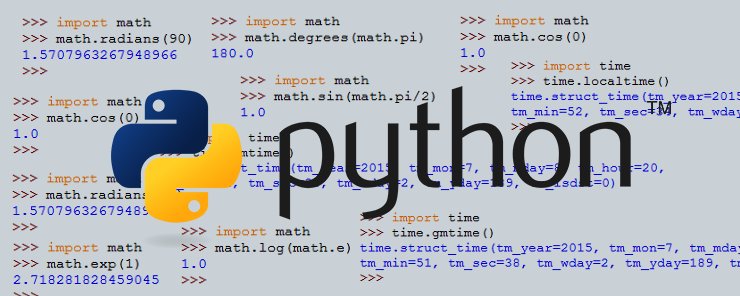In our previous article (Part 6 – Loops in Pythons) we seen different types of loops like for loop, while loop, do while loop and their working in Python. Today in this tutorial we will look upon different types of functions in Python.

What are functions?

A function is a named portion of code that achieves a specific task.

Why functions?

When a task is needed to be performed several times throughout a program, we have two choices:

• Copy the code that achieves the task, and paste it everywhere the task is to be performed.
• Declare a function with a name, and body that contains the required code. Wherever the task is needed, the function is called.

Okay, what is the problem in copying and pasting the same code several times? It is not a big deal!! So, why do I have to care about functions and such complications?!

Well, consider you have detected a syntax error in the code that performs the task; you will have to make the necessary corrections everywhere the code exists. Now, what if a need arises in the future to modify the code to add some new features or make some enhancements? Again, you will have to make the modifications everywhere the code exists. Besides being an error-prone process, it is also considered a very poor programming approach.

How Functions work?

Functions receive input (arguments), make the required processing, and finally return an output.

What are the Functions types?

There are two types of function:

• Built-in Functions
• User-Defined Functions

Built-in Functions

As the name implies, a built-in function is a predefined function that is ready for use. We have already encountered some instances for this type in our previous articles. We have used print, eval, input, factorial, type, and range. All we had to do in order to use those functions was just calling the required function, and passing arguments to them.

Mathematical Functions

Python has a rich list of mathematical functions that belong to the math package. In this section, we will discuss some of the important mathematical functions illustrated by examples.

```pow(x, y)
```

raises x to the power of y (equivalent to x**y)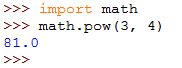```sqrt(x)
```

Calculates the square root of a positive number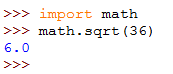```exp(x)
```

Raises the constant e to the power of x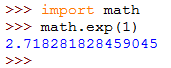```log(x)
```

Calculates the Natural Logarithm of the number x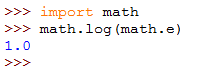```radians(x)
```

Converts from degrees to radian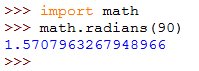```degrees(x)
```

Converts from radians to degrees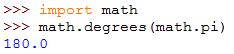```sin(x)
```

Calculates the sine of the angle x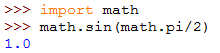```cos(x)
```

Calculates the cosine of the angle x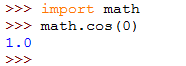* * * * * *

Random Number Generators

There will be cases when you need random numbers. One example for such cases is when you want to generate a random password. The package random contains a set of functions that help generate random numbers. In this section, we will see the function randint.

```randint(x,y)
```

The function randint returns a random integer in the range [x, y], including both x and y.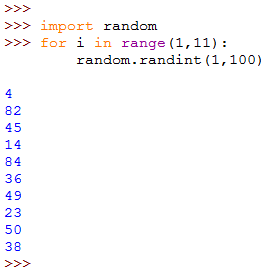We started by importing the random module. This is necessary to be able to use the functions defined in this module. To generate 10 random integers, we used a for loop that loops on the range between 1 and 11, where the upper bound of the range (11) is not counted. Each iteration executes the function randint(1, 100) to get a random number from the 1 to 100 inclusively.

* * * * * *

Time Functions

The time module contains a set of useful time-related functions. In this section, we will have a look on three functions with simple examples.

```time.gmtime()
```

Returns the full date and time info for the current GMT time.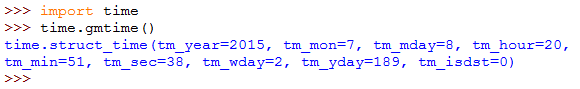```time.localtime()
```

Returns the current date and time for the local timezone.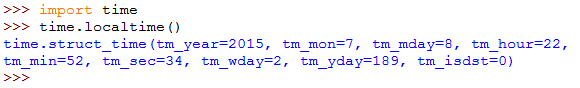```time.sleep(sec)
```

The sleep function delays the execution for the specified number of seconds.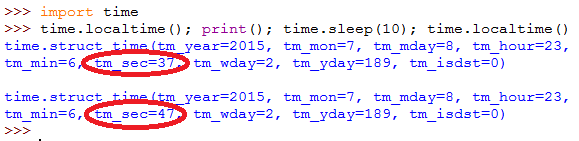* * * * * *

User-Defined Functions

The following is the syntax used to declare a function:

```def   functioname (arguments_list)
function_body
```

Example

The following script defines a function called delay. The function that accepts an integer number as its only argument, when called prints a message that it will sleep for a specific number of seconds. After sleeping this period, it prints another message that it is back after sleeping.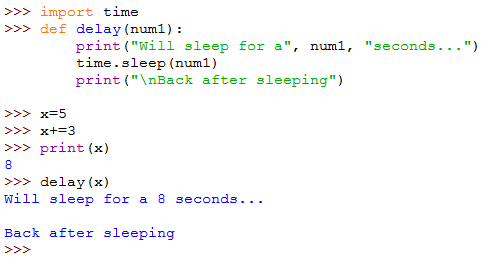Example

The following script calculates the area and perimeter of a circle given its radius as the user input.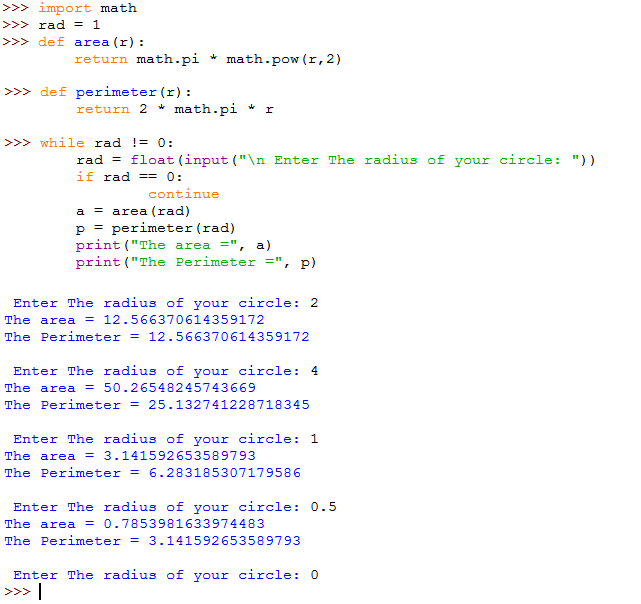Image 1 : A script  that defines two functions to calculate the area and perimeter of circles.

Example

The following script accepts two numbers from the user, and prints the greater one.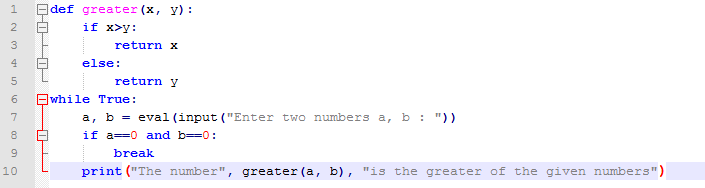Image 2: A script that calls a function to determine the greater of two numbers.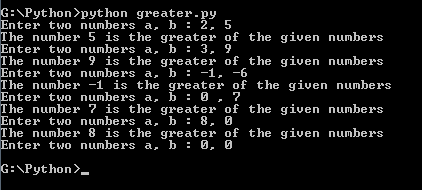Image 3: Executing the script.

Now, let’s explain things:

• Line 1: defines the function using the keyword def followed by the function name, then a colon (:)
• Lines 2 through 5: are the function body. The function checks if the first argument x is greater than the latter y; if so, the function returns x as the greater of the two numbers. If not, y is returned.
• Line 6: starts an infinite loop (while True:) that keeps prompting the user to enter two numbers (Line 7)
• Line 8: uses the if statement to check if both x and y equal 0. If so, the break statement (Line 9) is executed to exit the infinite loop.
• Line 10: calls the greater() function from inside the print function that prints a message to the user telling the greater number.

Simple, and Logic, isn’t it?!

* * * * * *

Default Arguments

Some functions have optional (default) arguments. When an argument is said to be optional, it means the function could work and achieve the required task whether this argument is passed to it or not.

In fact, this is not magic!! The idea is that behind the scene, in the function definition, that specific argument is declared and assigned a default value. So, we can write a function that takes:

• Only mandatory arguments.
• Only optional arguments.
• A mix of both mandatory and optional arguments, or
• No arguments at all.

There is only one restriction to using a mix of mandatory and optional arguments, is that the optional arguments must come after all the mandatory arguments.

Let’s simplify the theoretical concept by this example.

Example

If you have worked on Linux, it is likely you know the command seq. As its name implies, seq prints a sequence of numbers (one per line) from start to end in steps of step. So, the command accepts three arguments: start, end, and the step. In its definition, if the start argument is not passed, it defaults to 1, and if the step is not provided, it is also considered to be 1. That sounds familiar!! Ah, they are optional arguments, okay!!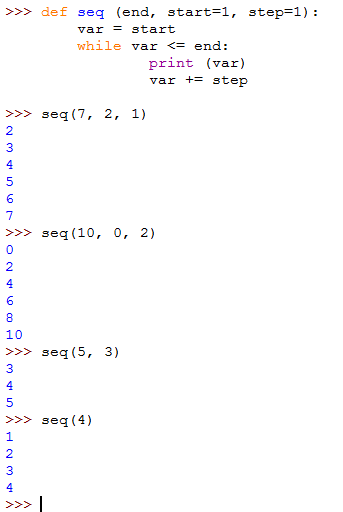Image 4: Defining and using the seq function using optional arguments.

You see that!! The function is declared with one mandatory argument, followed by two optional arguments (because they are initialized with default values). So, when the function is called, you have the choice to pass three, two, or only one argument to it. Interesting!!

To this point, we have talked about functions: what they are, why to use them, and how. We have seen that there are two main types of functions, built-in and user-defined. We have talked about both types, and supported the theoretical concepts by illustrative examples.

I hope you find this article useful.

Read More: Best C/C++ IDEs & Editors for Linux Revealed!

Previous articleLearn About Views in Zend Framework
Next articleCalendar Class in Zend Framework